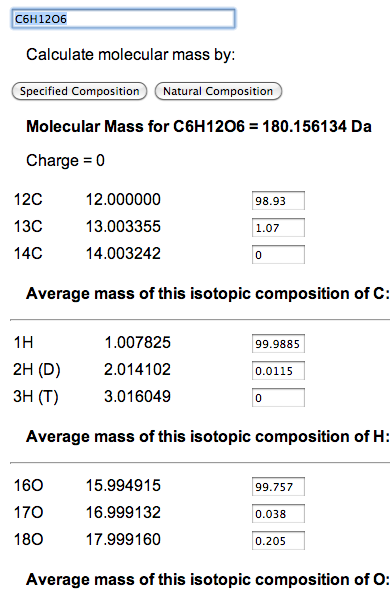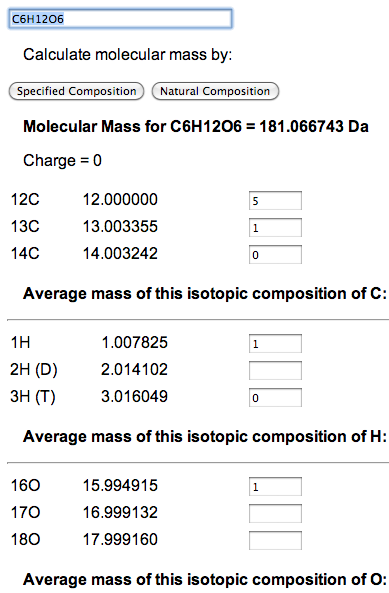## Pointers for Using the Molecular Mass Calculator

A user can enter the formula for the compound of interest, say C6H12O6, and click on the button that says 'Natural Composition'. This will compute the molecular mass of the compound at its natural isotopic composition, as well as the combinations of 12C, 13C, 14N, and 15N. There are also inputs for isotopic adjusting ratios by hand, which are initially set to natural abundance percentages as obtained from the National Institute of Standards and Technology (NIST).

Though natural abundance mass is useful when measuring a source to mix a sample, it is not a measurement that will be seen in a mass spectrometer. However, there is no reason why the abundance of an isotope needs to be expressed as a percentage. For example, Carbon lists three isotopes - 12C, 13C, and 14C - along with the corresponding percentages of 98.93, 1.07, and 0. To determine the weight of glucose (C6H12O6) where one atom is 13C and the rest are 12C, enter 5 for 12C and 1 for 13C. Then click 'Specified Composition'.

The ratios of isotopes are determined by a simple sum and divide. You can enter whole numbers, percentages, or decimal point numbers. Unfortunately, it will not calculate fractions. A blank field is considered zero. The easiest way to set all atoms of a particular element to a single isotope is to set field for that isotope to 1 and delete the others.

Calculation using natural isotopic compositionCalculation for 12C5 13C 1H12 16O6